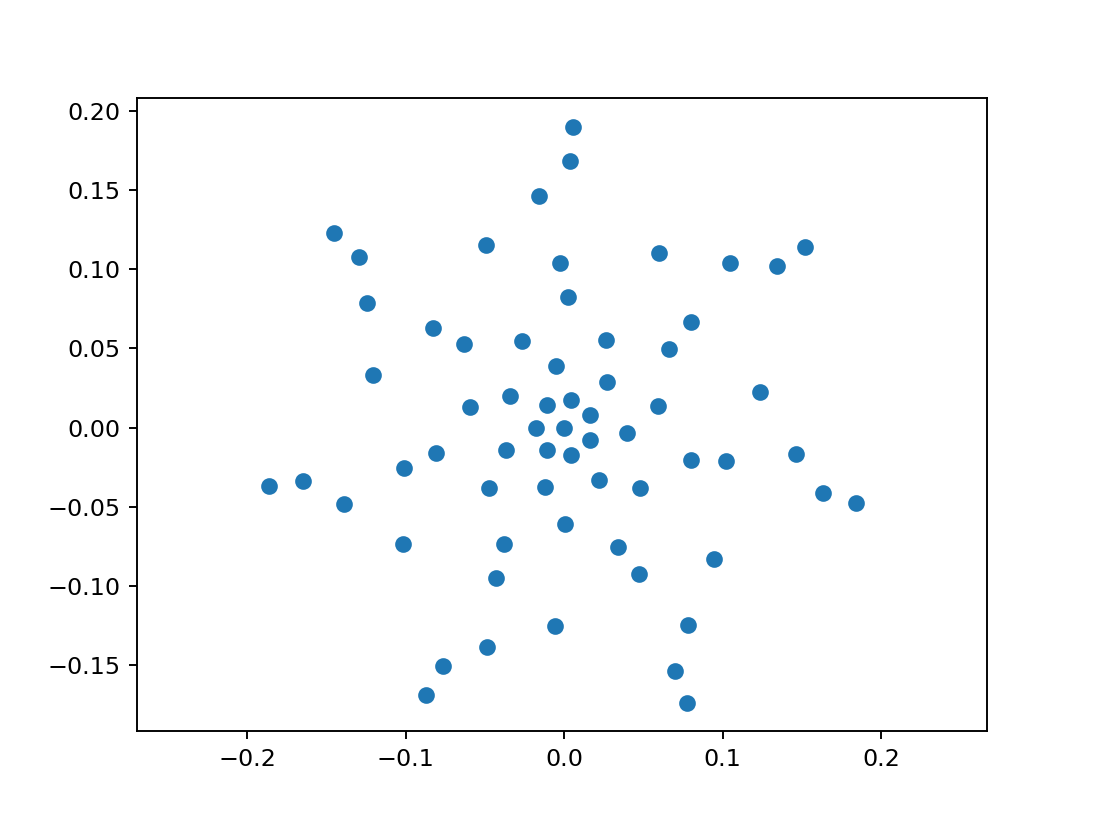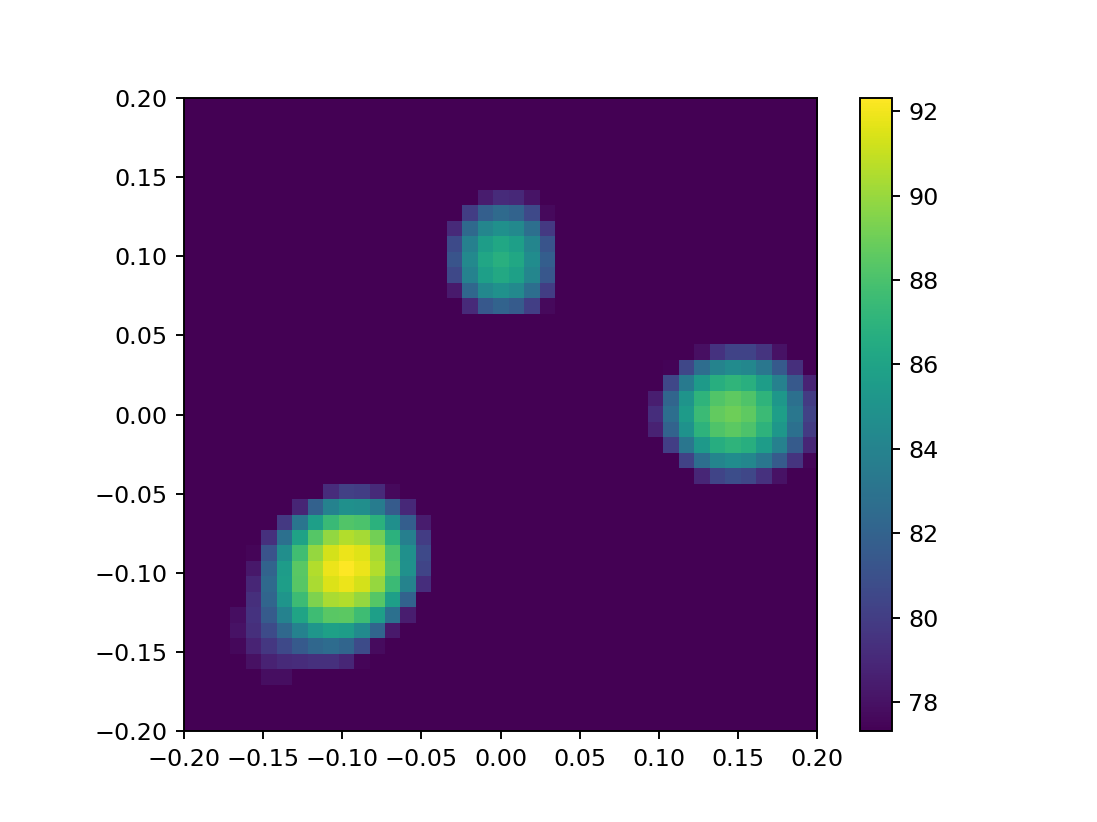This is the first in a series of three blog posts about the basic use of Acoular. It explains some fundamental concepts and walks through a simple example.

Acoular is a Python library that processes multichannel data (up to a few hundred channels) from acoustic measurements with a microphone array. The focus of the processing is on the construction of a map of acoustic sources. This is somewhat similar to taking an acoustic photograph of some sound sources.

To use Acoular, we first have to import Acoular into our notebook.

import acoular


In this example, we want to analyze time histories from 64 microphones stored in the file "three_sources.h5". The file contains data from a measurement (actually a simulated measurement in this special case) of scene with three different sound sources.

This file is in HDF5 format, which is an open all purpose numerical data container file format. Besides the time histories it contains information about the sampling rate. To learn about the internal structure of the file, have a look at the file! You will need an HDF5 file viewer (e.g. https://www.hdfgroup.org/downloads/hdfview/). For now, we can skip this step and simply load the file into our notebook. The file can be found in the same directory as the notebook itself.

ts = acoular.TimeSamples( name="three_sources.h5" )


The file is not directly opened using Python commands, but instead we use the TimeSamples class from Acoular. This class manages the data in the file in an intelligent way which even allows to use huge data files that do not fit into the memory.

If we inspect the ts object, we see not the data itself, but just the type and the location in memory.

ts

<acoular.sources.TimeSamples at 0x7ff0b3e48710>

We can use this object now to answer some questions about the data:

• How many channels,
• and how many samples do we have?
• What is the sampling frequency?
print( ts.numchannels, ts.numsamples, ts.sample_freq )

64 51200 51200.0


The signal processing can either take place in the time domain or in the frequency domain. To work in the frequency domain, the time history data must be transformed into power spectra. More specifically, we need the cross spectral matrix (CSM) which contains the pairwise cross spectra of all possible combinations of two channels. The cross spectral matrix is computed using Welch's method. For this, an FFT block size and a window type have to be chosen.

ps = acoular.PowerSpectra( time_data=ts, block_size=128, window="Hanning" )
ps.fftfreq()

array([    0.,   400.,   800.,  1200.,  1600.,  2000.,  2400.,  2800.,
3200.,  3600.,  4000.,  4400.,  4800.,  5200.,  5600.,  6000.,
6400.,  6800.,  7200.,  7600.,  8000.,  8400.,  8800.,  9200.,
9600., 10000., 10400., 10800., 11200., 11600., 12000., 12400.,
12800., 13200., 13600., 14000., 14400., 14800., 15200., 15600.,
16000., 16400., 16800., 17200., 17600., 18000., 18400., 18800.,
19200., 19600., 20000., 20400., 20800., 21200., 21600., 22000.,
22400., 22800., 23200., 23600., 24000., 24400., 24800., 25200.,
25600.])

We see that after the FFT we do have spectra with 400 Hz frequency spacing. For most applications this would be too coarse. We use it here to get faster processing. If we wish to have finer spacing, we need larger block sizes.

Up to now we have defined how the processing should be done, but we did not compute the actual CSM. Nearly all expensive computations in Acoular are only done on demand using a lazy evaluation paradigm. We can trigger the computation of the cross spectral matrix by just asking for it. In this example we do want to print this already large matrix. Instead we only print its shape.

ps.csm.shape

[('three_sources_cache.h5', 1)]

(65, 64, 64)

This matrix actually has the dimensions 65 (number of frequencies) times 64 by 64 (number of microphone channels).

To continue with our task to generate an acoustic photograph, we need the microphone positions. In Acoular, one option is to read them from an XML file. The file looks like this:

<?xml version="1.0" encoding="utf-8"?>
<MicArray name="array_64">
<pos Name="Point 1" x="0.152" y="0.1141" z="0"/>
<pos Name="Point 2" x="0.134" y="0.1021" z="0"/>
...
<pos Name="Point 64" x="0.0218" y="-0.0329" z="0"/>
</MicArray>


(most of the lines are omitted here)

A MicGeom object handles the file:

mg = acoular.MicGeom( from_file="array_64.xml" )
mg.mpos

array([[ 0.152 ,  0.134 ,  0.1043,  0.0596,  0.0798,  0.0659,  0.0262,
0.0272,  0.    ,  0.004 ,  0.0162,  0.0162,  0.004 , -0.0112,
-0.018 , -0.0112, -0.145 , -0.1294, -0.1242, -0.1209, -0.0828,
-0.0631, -0.0595, -0.034 ,  0.0056,  0.0037, -0.016 , -0.0492,
-0.0024,  0.0022, -0.0267, -0.0054, -0.0874, -0.0764, -0.049 ,
-0.0058, -0.0429, -0.0378,  0.0003, -0.0121, -0.1864, -0.1651,
-0.1389, -0.1016, -0.1008, -0.0809, -0.0475, -0.0369,  0.1839,
0.1634,  0.146 ,  0.1235,  0.1019,  0.0799,  0.0594,  0.0393,
0.0774,  0.0697,  0.0778,  0.0944,  0.0473,  0.0338,  0.0478,
0.0218],
[ 0.1141,  0.1021,  0.1036,  0.1104,  0.0667,  0.0497,  0.0551,
0.0286,  0.    , -0.0175, -0.0078,  0.0078,  0.0175,  0.0141,
0.    , -0.0141,  0.1228,  0.1079,  0.0786,  0.0335,  0.0629,
0.0531,  0.0133,  0.0202,  0.1899,  0.1685,  0.1461,  0.1155,
0.104 ,  0.0825,  0.0548,  0.0391, -0.1687, -0.1502, -0.1386,
-0.1254, -0.0947, -0.0733, -0.061 , -0.0376, -0.0368, -0.0339,
-0.0481, -0.0736, -0.0255, -0.0162, -0.0382, -0.014 , -0.0477,
-0.0411, -0.0169,  0.0223, -0.0208, -0.0205,  0.0138, -0.0034,
-0.1735, -0.1534, -0.1247, -0.0827, -0.0926, -0.0753, -0.0378,
-0.0329],
[ 0.    ,  0.    ,  0.    ,  0.    ,  0.    ,  0.    ,  0.    ,
0.    ,  0.    ,  0.    ,  0.    ,  0.    ,  0.    ,  0.    ,
0.    ,  0.    ,  0.    ,  0.    ,  0.    ,  0.    ,  0.    ,
0.    ,  0.    ,  0.    ,  0.    ,  0.    ,  0.    ,  0.    ,
0.    ,  0.    ,  0.    ,  0.    ,  0.    ,  0.    ,  0.    ,
0.    ,  0.    ,  0.    ,  0.    ,  0.    ,  0.    ,  0.    ,
0.    ,  0.    ,  0.    ,  0.    ,  0.    ,  0.    ,  0.    ,
0.    ,  0.    ,  0.    ,  0.    ,  0.    ,  0.    ,  0.    ,
0.    ,  0.    ,  0.    ,  0.    ,  0.    ,  0.    ,  0.    ,
0.    ]])

The mg object now contains all information about the microphone positions. Now let us this to plot the microphone geometry. For plotting we use the matplotlib package.

%matplotlib notebook
import matplotlib.pylab as plt

plt.plot( mg.mpos, mg.mpos, 'o' )
plt.axis( 'equal' );This gives a nice impression of the microphone arrangement which consists of 7 inertwined planar logarithmic spirals (hard to see the spirals, I admit).

To map the sound, we need a mapping grid. Here we construct a simple regular and rectangular grid. Note that we have to decide about the size, spacing and the distance (z coordinate) from the array. Size and spacing define the number of points in the grid. The more points in the grid, the better the resolution of the acoustic photograph, but the longer the processing will take.

rg = acoular.RectGrid( x_min=-0.2, x_max=0.2,
y_min=-0.2, y_max=0.2,
z=0.3, increment=0.01 )
rg.pos()

array([[-0.2 , -0.2 , -0.2 , ...,  0.2 ,  0.2 ,  0.2 ],
[-0.2 , -0.19, -0.18, ...,  0.18,  0.19,  0.2 ],
[ 0.3 ,  0.3 ,  0.3 , ...,  0.3 ,  0.3 ,  0.3 ]])

The rg object now has all information about the grid.

The actual method we will use is beamforming. Basically this works by using the combined microphones as a sound receiver with a directivity that is steered to each one of the grid points in turn. One important element in beamforming and similar methods is the steering vector implemented in Acoular in the SteeringVector class. This vector "connects" grid and microphones and takes into account the environmental conditions. These conditions are defined by the speed of sound and a possible background flow. If not set explicitely, a 'standard' environment is created in Acoular which assumes quiescent conditions (no flow) and a speed of sound of 343 m/s (air at 20°C).

st = acoular.SteeringVector( grid=rg, mics=mg )
st.env.c

343.0

Indeed the standard speed of sound is used.

Now, we define the method we want to use and set up a standard (basic) beamformer. For this need two ingredients: the CSM and the steering vector.

bb = acoular.BeamformerBase( freq_data=ps, steer=st )


Remember that Acoular uses lazy evaluation. No computation yet!

This means although we set up everything needed to perform beamforming, computation is postponed until the results are actually needed.

This will happen if we ask for the result. In this example we are interested in the sum for of all FFT frequency lines in the 3rd octave band 8000 Hz. This means we need the maps for all these frequencies and then add them together to synthetically "mimic" the result of a third octave filter for that band. The result is given as mean square sound pressure contribution at the center location of the microphone array. In Acoustics, we are usually like to have the results in the form of sound pressure levels (SPL). Acoular has a helper function L_p to compute the levels from the mean square.

pm = bb.synthetic( 8000, 3 )
Lm = acoular.L_p( pm )

[('three_sources_cache.h5', 2)]


The map is now stored in the array variable pm and the array Lm holds the soundpressure levels computed from this.

print(Lm.shape)
print(Lm)

(41, 41)
[[-350.         -350.         -350.         ... -350.
-350.         -350.        ]
[-350.         -350.           61.5185086  ... -350.
-350.         -350.        ]
[  66.4266366    65.93676977   68.3501483  ... -350.
-350.         -350.        ]
...
[-350.         -350.         -350.         ... -350.
-350.         -350.        ]
[-350.           54.71250037   46.46415942 ...   48.39371023
58.52533737 -350.        ]
[  58.13562703   62.27400964   61.09820493 ... -350.
61.88124663   61.96166613]]


The map has the same dimensions (41 x 41) as the grid. Any zero result in the map will be clipped to -350 dB level instead of -infinity.

Now lets plot the map as a color-coded image with 15 dB dynamic range between both ends of the color scale.

plt.figure()
plt.imshow( Lm.T, origin="lower", vmin=Lm.max()-15, extent=rg.extend() )
plt.colorbar();Now we enjoy the acoustic photograph of the three sources. Although it looks a bit blurry, we can guess the location of the sources and see as well that the sources have different strength.

This post has demonstrated how to use Acoular to produce a sound map or acoustic photograph with beamforming. Here you can see how to change some parameters and how to use different methods in the time domain and here you can learn about Acoular and time domain processing.